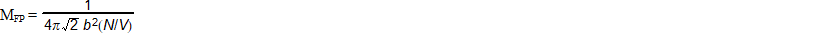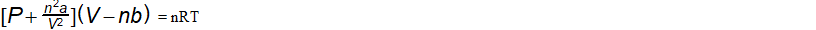### AP Physics 1 Week 9 (Aug 06 - Aug 12, 2017)

Part 1:  Algebra (Rearrange)

1.  solve for b2.  solve for N3.  solve for V4.  solve for n5.  solve for VPart 2:  Algebra (Inverse and direct relationships)

6) The electric current I, is amperes, in a circuit varies directly as the voltage V.  When 12 volts are applied, the current is 4 amperes.  What is the current when 18 volts are applied?

7)  The volume V of gas varies inversely to the pressure P.  The volume of a gas is 200 cm3 under pressure of 32 kg/cm2.  What will be its volume under pressure of 40 kg/cm2?

8) The number of kilograms of water in a person’s body varies directly as the person’s mass. A person with a mass of 90 kg contains 60 kg of water. How many kilograms of water are in a person with a mass of 50 kg?

9) On a map, distance in km and distance in cm varies directly, and 25 km are represented by 2cm. If two cities are 7cm apart on the map, what is the actual distance between them?

10) The time it takes to fly from Los Angeles to New York varies inversely as the speed of the plane. If the trip takes 6 hours at 900 km/h, how long would it take at 800 km/h?

Part 3: Geometry/Trigonometry
(For Physics, make sure your calculator is set in degrees)

Right Triangle Review:
Pythagorean Theorem (math version) c2 = a2 + b2, (physics version) r2 = x2 + y2
r = hypotenuse of the right triangle
x = horizontal or (east-west) component of the triangle
y = vertical or (north-south) component of the triangle
q = the reference angle always between the x axis and the hypotenuse

horizontal component x = r*cos(q)  vertical component y = r*sin(q)

The following problems use compass directions that start from the north and go clockwise.  North is a compass direction of 0 degrees, east is a compass direction of 90 degrees, south is a compass direction of 180 degrees, and west is a compass direction of 270 degrees.  Continuing back to north, is a compass measure of 360 degrees.  (In your math class, you have been taught the Babylonian unit circle based on the path of the sun moving across the sky.  The sun appears in the east or 0 degrees, then counter-clockwise, it passes it's zenith at a line perpendicular to the north or 90 degrees, and finally sets in the west at the end of each day at 180 degrees.  To complete the circle, south is defined at 270 degrees, while back to east is 360 degrees.) Both compass and Babylonian directional angles are given.

11.  A plane flies on a course 25 degrees north of east (compass: 65 degrees, Babylonian: 25 degrees) at 540 miles per hour.  How many miles north of its starting point will it be at the end of an hour and a half?
(DBW, p397, #3)

12(a), 13(b) A river flows from north to south.  To cross from the east bank directly to the west bank a boat captain finds that he must keep a course of 14 degrees north of west (compass: 284 degrees, Babylonian: 166 degrees).  If the trip takes fifteen minutes when the boat travels 12.4 miles per hour, (a) how wide is the river, and (b) how swift is the current?   (DBW, p397, #7)

14.  A plane's airspeed is 400 miles per hour on a heading of 45 degrees south of east (compass: 135 degrees, Babylonian 315 degrees).  If a wind is blowing from the west with a speed of 50 miles per hour, what is the ground speed and direction of the plane?
(DBW, p438, #1)

15.  A ship sails 20 miles on a course of 55 degrees north of east (compass: 35 degrees, Babylonian 55 degrees), and then sails 30 miles on a course 10 degrees south of east (compass: 100 degrees, Babylonian: 350 degrees).  How far and in what direction is the ship from the starting point.  (DBW, p438, #5)

Part 4:  Word Problems

16. The altitude h of an object t seconds after being thrown vertically upward with a speed of v feet per second from an altitude of k feet is given by the formula:  h = -16t2 + vt + k . Determine when a ball tossed upward with a speed of 48 feet per second from a terrace 300 feet above the ground will be 236 feet above the ground.  (DBW, p135, example 3)

17. Using the equation from #16:  A missile is fired from ground level with an upward velocity of 6400 feet per second.  When will it return to the ground?  (DBW, p137, #5)

18. Using the equation from #16:  How much time will elapse before a missile fired with an upward velocity of 8000 feet per second from the edge of a cliff 1300 feet high again reaches an altitude of 1300 feet? (DBW, p137, #6)

19. Using the equation from #16:  From an airplane whose altitude is 2064 feet a bullet is fired with an upward muzzle velocity of 3200 feet per second.  When will the bullet's altitude be 8400 feet?  (Explain why there are two times for the solution.)

(DBW, p138, # 19)

20. Using the equation from #16:  When will a ball thrown with a downward velocity of 64 feet per second from the roof of a building 720 feet high hit the ground?  (Hint, the initial velocity v will have to be -64 feet per second.) (DBW, p175, #10)